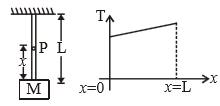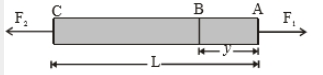You’ve reached the end of your free Videos limit.
#7-Solved-Examples-1
(Physics) > Laws of Motion
Unable to watch the video, please try another server
Related Practice Questions :

A plastic box of mass 5 kg is found to accelerate up at the rate of g / 6, when placed deep inside the water. How much sand should be put inside the box so that it may accelerate down at the rate of g / 6?

1.  2kg

2.  3kg

3.  4kg

4.  5kg

High Yielding Test Series + Question Bank - NEET 2020

Difficulty Level:

Tension on the string at point P is T. The graph for tension (T) versus x is shown in the figure. Then the string is:1.  massless

2.  massfull

3.  tension on every point on the string is same when the string is having finite mass 4

4.  None of these

High Yielding Test Series + Question Bank - NEET 2020

Difficulty Level:

A rod of length L and mass m is acted on by two unequal forces ${F}_{1}$ and ${F}_{2}\left(<{F}_{1}\right)$ as shown in the following figureThe tension in the rod at a distance y from the end A is given by :

1. ${F}_{1}\left(1-\frac{y}{L}\right)+{F}_{2}\left(\frac{y}{L}\right)$

2. ${F}_{2}\left(1-\frac{y}{L}\right)+{F}_{1}\left(\frac{y}{L}\right)$

3. $\left({F}_{1}-{F}_{2}\right)\frac{y}{L}$

4. None of the above

High Yielding Test Series + Question Bank - NEET 2020

Difficulty Level:

Five persons A, B, C, D & E are pulling a cart of mass 100 kg on a smooth surface and the cart is moving with acceleration 3 $\mathrm{m}/{\mathrm{s}}^{2}$ in east direction. When person 'A' stops pulling, it moves with acceleration 1 $\mathrm{m}/{\mathrm{s}}^{2}$ in the west direction. When person 'B' stops pulling, it moves with acceleration 24 $\mathrm{m}/{\mathrm{s}}^{2}$ in the north direction. The magnitude of the acceleration of the cart when only A & B pull the cart keeping their directions same as the old directions are:

1.  26 $\mathrm{m}/{\mathrm{s}}^{2}$

2.

3.  25 $\mathrm{m}/{\mathrm{s}}^{2}$

4.  30 $\mathrm{m}/{\mathrm{s}}^{2}$

High Yielding Test Series + Question Bank - NEET 2020

Difficulty Level:

A coin is dropped in a lift. It takes time t1 to reach the floor when lift is stationary. It takes time t2 when lift is moving up with constant acceleration. Then

(1) t1 > t2

(2) t2 > t1

(3) t1 = t2

(4) t1 >> t2